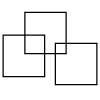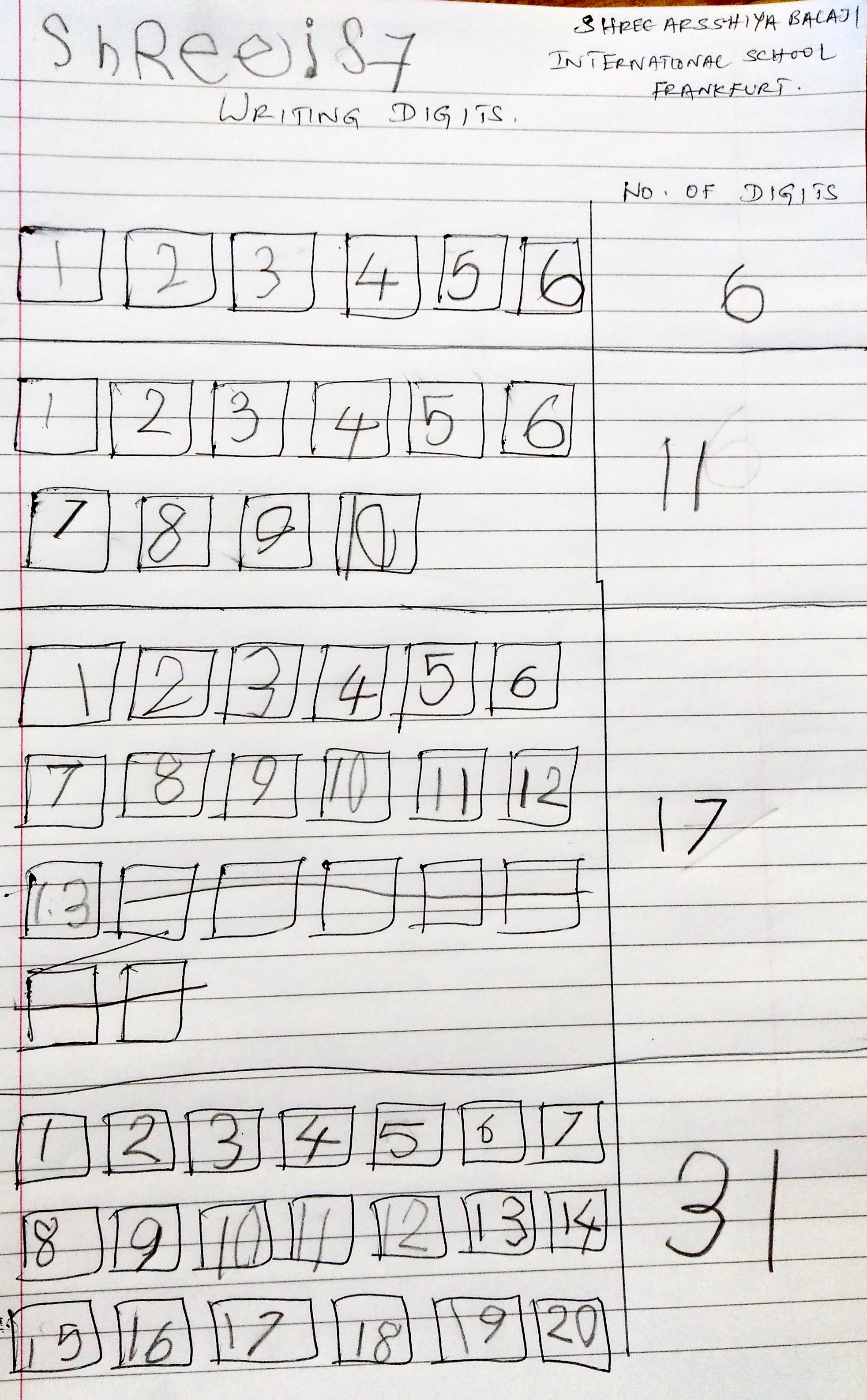#### You may also like### Three Squares

What is the greatest number of squares you can make by overlapping three squares?### Two Dice

Find all the numbers that can be made by adding the dots on two dice.### Biscuit Decorations

Andrew decorated 20 biscuits to take to a party. He lined them up and put icing on every second biscuit and different decorations on other biscuits. How many biscuits weren't decorated?

# Writing Digits

##### Age 5 to 7 Challenge Level:

Junsu from Ms. Brown's class at The Bangkok Patana School in Thailand sent in the following:

The numbers under 10 have a single digit, so when you write it down it counts as writing one digit.
Numbers starting from 10 have two digits, so it counts as writing two digits.
From 1-9 it is 1 x 9 = 9 digits
She wrote a total of 17 digits so 17 - 9 = 8. She has eight digits left, and from 10-20 each number counts as writing two digits 8/2=4, so she wrote four two-digit numbers which is 13

If she writes the numbers up till 20,  1 to 9 = 9 digits,  10 to 20 = 2 x 11 = 22 digits
22+9=31,  total 31 digits    Answer: 31

Hakuya from The Maadi British International School in Egypt sent in his thoughts:

I started by writing the numbers and then counting the digits.  I noticed that the 17th digit meant that Lee finished at the number 13. I then carried on writing to 20 and counted the digits again, there were 31 digits.
The problem asked me to think of a way of working it out without writing the numbers. I decided that you should count in 1's to 9 and then count in 2's.  So number 10 would have 11 digits.

Majid from Essex Primary School wrote this very thorough account:

Well, in order to figure this out, you need to know your numbers. The first two questions are easy to figure out. The next number after 5 is 6 as the sequence's rule is +1.
Writing seventeen digits doesn't necessarily mean the number she stopped at is 17. The question asks how many digits. In that case, 1-9 is 9 digits as they don't have more than one digit in their number. So, 17 take away 9 is 8. If numbers up to 99 have two digits, the number will not go that high as we only have 8 more digits. 8 divided by 2 is 4. So four more numbers. 10, 11, 12,13. The number she stopped at is 13.

The next question, how many digits up until 20? Well, we know seventeen digits are up until 13. So 13-20 is seven numbers. We know the numbers are two-digit numbers, 7 x 2 is 14 so fourteen digits from 13-20 (not including 13). 14 + 17 is 31. So thirty-one digits up until 20.

You don't need to count the digits one by one to work it out. But of course, if you find it easier to do so, it's all right. Another way to work it out is knowing how many one-digit numbers are included, how many two-digit numbers are included and so on. Knowing that you have to multiply the number of numbers by how many digits it has.

An example:
1-200.
1-9 is nine digits. Nine numbers times 1= 9
10-99 is 90 numbers (including 10 and 99). So 90x2 is 180. So 180 digits.
100-200 is 101 numbers (Including 100 and 200). So 101x3 is 303 digits.
9+180+303=492. Between 1 and 200 (including 1 and 200), there are 492 digits.

I found a file, an answer from the internet to prove my answer.

Shree from The International School Frankfurt, Germany sent in a picture of her work:Thanks for these really good examples of how it may be worked out.